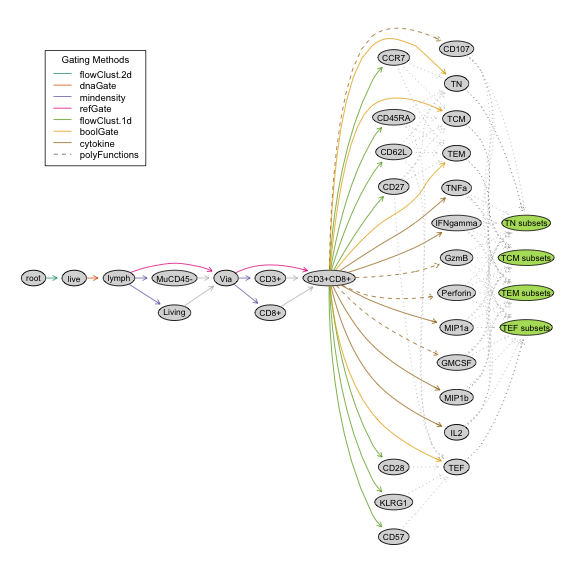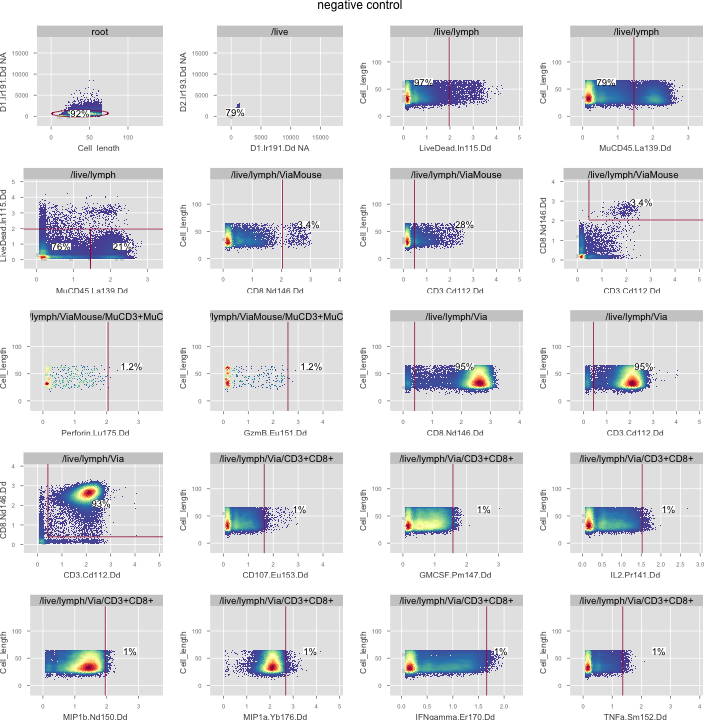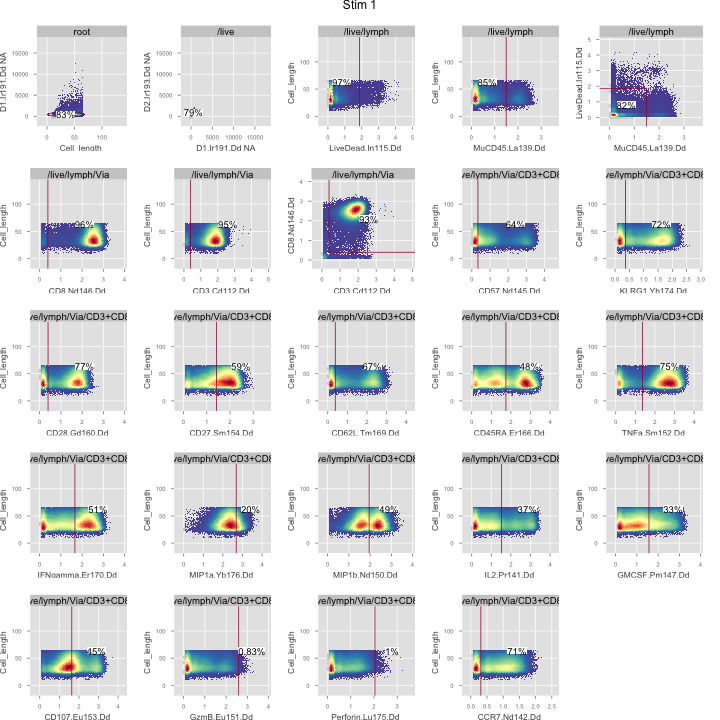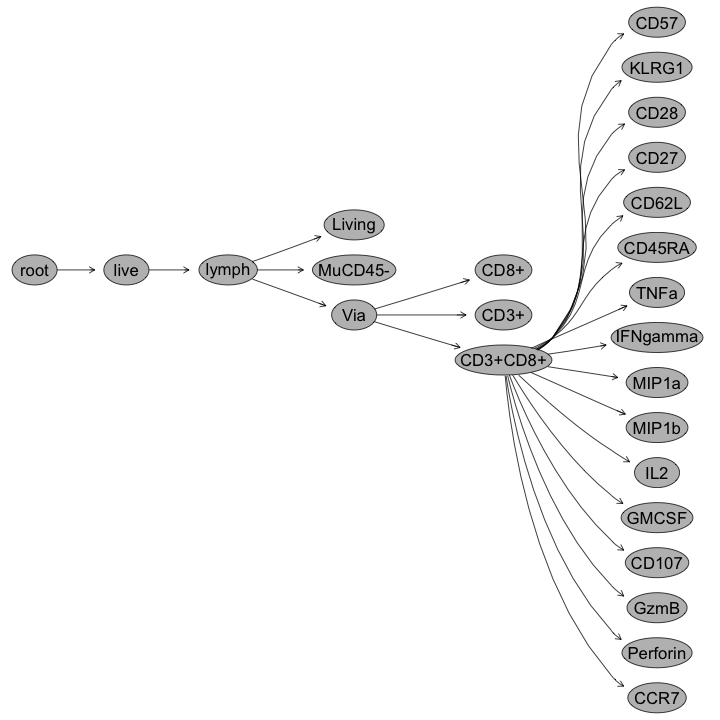View on GitHub

# OpenCyto

## A Robust BioConductor Framework for Automated Flow Data Analysis

OpenCyto Gating of SDY207

# OpenCyto Gating of SDY207

Data from Newell EW, Sigal N, Bendall SC, Nolan GP, Davis MM. Cytometry by Time-of-Flight Shows Combinatorial Cytokine Expression and Virus-Specific Cell Niches within a Continuum of CD8+ T Cell Phenotypes. Immunity. 2012.

Here we re-analyze several of the samples using an OpenCyto gating pipeline.

### Availablility

This script and others, as well as data for reproducing this analysis can be downloaded from here

### Set up

First, we load required R libraries, define our working directory and output directory. These should be customized.

# Load libraries
library(openCyto)
library(flowCore)
library(data.table)
library(mvtnorm)
library(grDevices)

# Output directory for figures and a working directorywhere the script and data are located.
# These should be customized.
OUTPATH<-"./"
WORKDIR<-"/Users/gfinak/Dropbox/GoTeam/Projects/Paper-OpenCytoPipeline/SDY207/reproducible/"
setwd(WORKDIR)


Next we define some useful functions for analysis. Since this is CyTOF data, we need a DNA/DNA gate, which is the CyTOF equivalent of gating on cells and excluding debris.

We define a custom gating function a wrapper for OpenCyto, and a function to transform the channels of the FCS files.

# A custom gating function for a DNA/DNA gate on CyTOF data.
# Finds the intersection between a quantile of a multivariate normal fit
# of a population and a boundary along y = -x+b
# author: jfreling@fhcrc.org
boundry <-  function(xs) {
# find the boundry events that are above a quantile and below a line

cxs <- scale(xs) # scale data so that it can be compaired to the results from qnorm
f <- qnorm(0.95) # set a boundry level
pd <- dmvnorm(c(f, f)) # and find the p(x) for that level

pxs <- dmvnorm(x=cxs)
idxs <- (pxs > pd) # find those points who are above the boundy level

idxs2 <- ((-1*cxs[,1]) + 1.96) > cxs[,2] # find points that are below the line with y=-1*x+b
pos_xs <- xs[idxs&idxs2,] # intersection of points below line and above threshold level

hpts <- chull(pos_xs) # find the boundry points of the intersection of cells
return(pos_xs[hpts,])
}

# Wrapper function for the boundary gate. This is registered by OpenCyto for use in the template.
.dnaGate <- function(fr, pp_res, xChannel=NA, yChannel=NA, filterId="", ...){
xs <- exprs(fr[,c(xChannel, yChannel)]) # extract just the parameter values being inspected
pnts <- boundry(xs) # find the verticies of the gate
return(polygonGate(boundaries=pnts, filterId=filterId))
}

# Transformation function (logicle transform).
transformx <- function(x) {
# take a data set and strip out unwanted channels, and apply logicle transform to it
lgcl <- logicleTransform(w=0.25, t=16409, m=4.5, a=0)
markers <- colnames(x)
markers <- markers[!grepl("Tet", markers)]
markers <- markers[!grepl("blank", markers)]
markers <- markers[!grepl("Ba138", markers)]
markers <- markers[!grepl("CD3", markers)]
markers <- c(markers,"CD3.Cd112.Dd")
x <- x[, markers]
markers <- markers[!grepl("DNA", markers)]
markers <- markers[!grepl("Cell_length", markers)]
lgcl <- logicleTransform(w=0.25, t=16409, m=4.5, a=0)
logicle_transform <- transformList(markers, lgcl)
x <- transform(x,logicle_transform)

return(x)
}


Next we register the new gating function with OpenCyto. The method depends on 'mvtnorm', and is a gating method, rather than a preprocessing method. The wrapper is .dnaGate and the method is referred to as dnaGate (without the dot) in the gating template.

registerPlugins(fun=.dnaGate,methodName='dnaGate', dep='mvtnorm','gating')

## Registered dnaGate


#### Reading and transforming the data, and constructing a GatingSet

We read in the FCS files, and transform the channels, then construct GatingSet objects.

#Use a negative control to establish cytokine levels then apply them to the two treatment samples

negfile.name <- "./data/E2D5NS_cells_found.fcs" #File location
neg <- read.FCS(negfile.name, transform=FALSE, alter.name=TRUE) #read it
neg <- transformx(neg) #our transformation function

neg_fs <- flowSet(c(neg)) #constrcut a flowSet
sampleNames(neg_fs)<-"negative control"
neg_gs <- GatingSet(neg_fs) #construct a GatingSet where we can add gates.

## gating negative control ...
## done!


Stimulated samples:

# load two samples to analyize and gate to the cytokine gates,
xfile.name <- "./data/E2D5_cells_found.fcs"
x <- read.FCS(xfile.name, transform=FALSE, alter.names=TRUE)
x <- transformx(x)

yfile.name <- "./data/E2D6_cells_found.fcs"
y <- read.FCS(yfile.name, transform=FALSE, alter.names=TRUE)
y <- transformx(y)
fs  <-  flowSet(c(x,y))
sampleNames(fs)<-c("Stim 1","Stim 2")
gs <- GatingSet(fs)

## gating Stim 1 ...
## gating Stim 2 ...
## done!


We load up the gating template for the negative control and the stimulatedsamples. There are no boolean cytokine gates for the negative control, only the marginals.

neg_gtFile <- "./template_tcell_control.csv"
gtFile <- "./template_tcell.csv"

neg_gtcell <-gatingTemplate(neg_gtFile)
gt_tcell <- gatingTemplate(gtFile)


Here's an example of the tempate for the stimulated sample.

plot(gt_tcell)#### Run the gating on the data

Apply the template to the negative control GatingSet, and save the gated data.

system.time(gating(neg_gtcell, neg_gs, mc.core = 4, parallel_type = "multicore"))

##    user  system elapsed
##   12.04    3.70   15.79


if(!file.exists("gating/open_cyt_neg_gs.dat")){
save_gs(neg_gs, 'gating/open_cyt_neg_gs.dat')
}


Next, gate the stimulated samples, stopping before the Effector T-cell cytokine subsets. We then prune the gating tree and add the marginal cytokine gates from the negative control to the CD8+/CD3+ subset, recompute statistics, and continue gating the boolean cytokine subsets.

# gate to the cytokine level
system.time(gating(gt_tcell, gs, mc.cores = 4, parallel_type = "multicore", stop.at='TEF subsets'))

##    user  system elapsed
##  231.78   20.94  265.25

#Remove the TEF subset
Rm("TEF",gs)

# copy the cytokine gates from the negative control and add them to the gatingset used on the treatment samples
for(i in list("TNFa","IFNgamma","MIP1a","MIP1b", "IL2", "GMCSF", "CD107", "GzmB", "Perforin")) {
try(Rm(i,gs)) #remove the old gate
gate <- getGate(neg_gs, i) #get the new one
add(gs,gate\$negative control, parent='CD3+CD8+') #add it
}

#Need to recompute where the gates have changed

# finish gating by generating the polyfunctional cytokine gates
system.time(gating(gt_tcell, gs))

##    user  system elapsed
##  222.49   59.18  273.33


# and save the results
if(!file.exists("gating/open_cyt_gs.dat")){
save_gs(gs, 'gating/open_cyt_gs.dat',overwrite=TRUE)
}


#### Visualization

We can plot the gates from each sample, as well as the gating tree.

The negative control:

plotGate(neg_gs[], default.y="Cell_length", xbin=0)A stimulated sample, with the cytokine gates from the negative control:

plotGate(gs[], default.y="Cell_length", xbin=0)

## skipping boolean gates!Boolean gates are not shown in the gating tree.

plot(gs[])